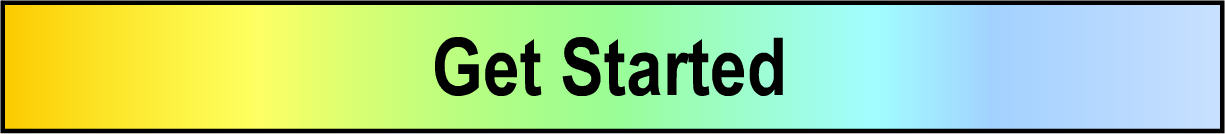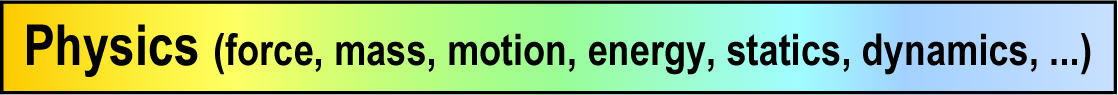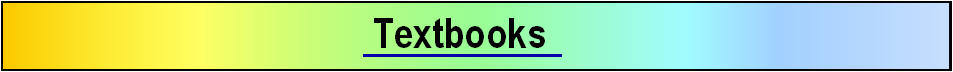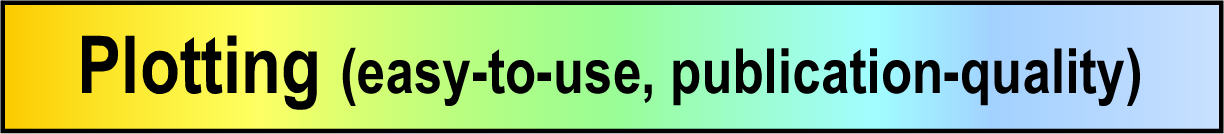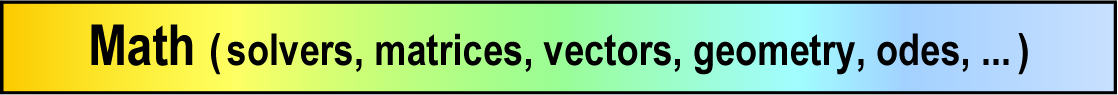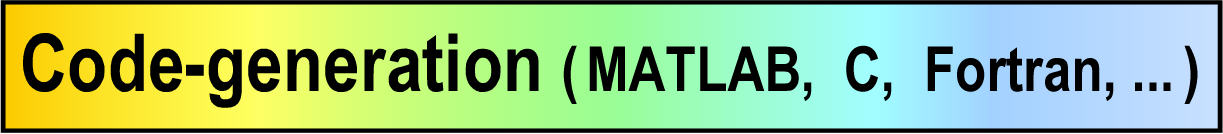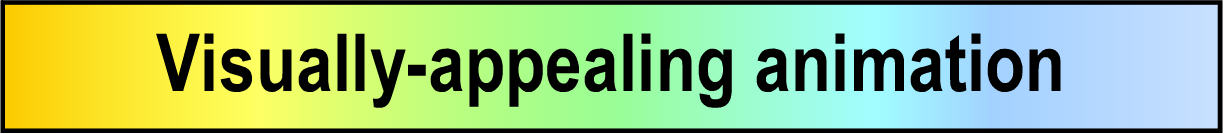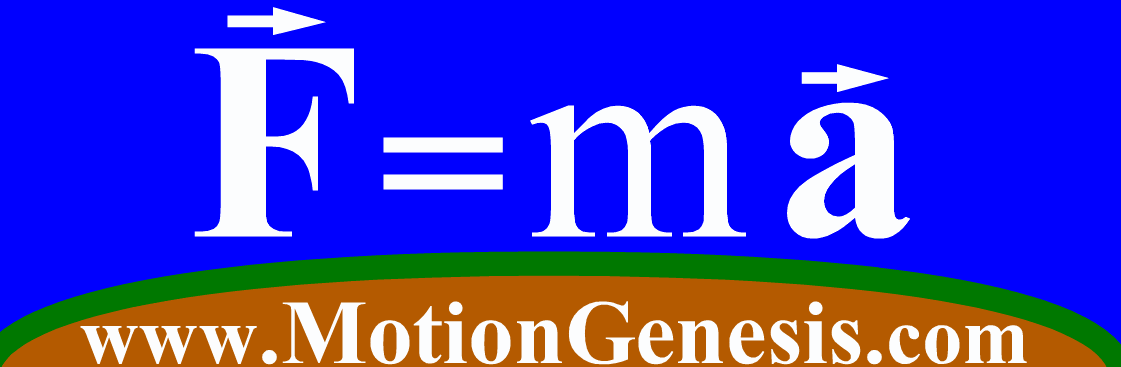MotionGenesis code generation Control systems integration (MATLABŽ)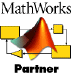MotionGenesis auto-generates   MATLABŽ, C, Fortran   codes, making it easy to:
• Modify integration parameters, initial values, constants, etc.
• Modify MATLABŽ plots created in response to the OutputPlot command.
• Modify .m files for event handling (e.g., detecting ground contact)
• Modify .m files for use with the MATLABŽ controls and optimization toolboxes.
• Run Monte-Carlo simulations to validate designs and test parameter sensitivity.

MotionGenesis code generation: MATLABŽ, C, Fortran
• Writes exceptionally fast and memory-optimized software programs.
• Writes easy-to-use and modify MATLABŽ, C, and Fortran codes.
• Automated code input/output and enhanced units and unit conversions.
• Improved algorithm for Code Nonlinear with MATLABŽ codes.
• Solves linear and nonlinear equations and sets proper initial values.
• Solves constrained systems with embedded or augmented constraints.
• Generates codes to solve coupled sets of linear/nonlinear algebraic and/or differential equations.
• Introduces intermediate variables for small compact code and reduced operations count.
• Symbolically pre-optimizes code for high-speed, low-memory, in-the-loop hardware.
• Incorporate numerical integration checking functions for monitoring simulation accuracy.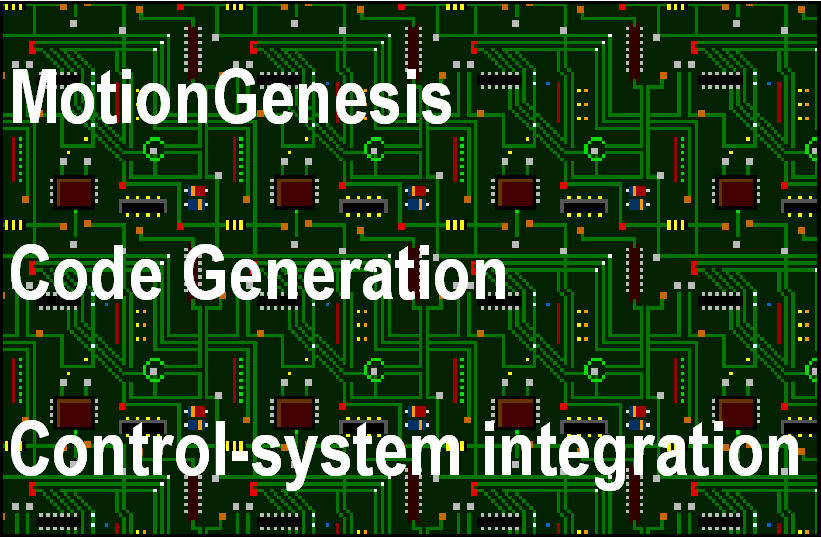Built-in vectors, geometry, physics, and code-generation